## Resource Allocation Graph-

Before you go through this article, make sure that you have gone through the previous article on Resource Allocation Graph.

We have discussed-

• Resource Allocation Graph (RAG) is a graph that represents the state of a system pictorially.
• There are two components of RAG- Vertices and Edges.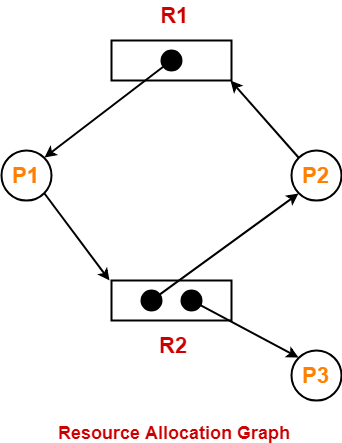Using Resource Allocation Graph, it can be easily detected whether system is in a Deadlock state or not.

The rules are-

## Rule-01:

In a Resource Allocation Graph where all the resources are single instance,

• If a cycle is being formed, then system is in a deadlock state.
• If no cycle is being formed, then system is not in a deadlock state.

## Rule-02:

In a Resource Allocation Graph where all the resources are NOT single instance,

• If a cycle is being formed, then system may be in a deadlock state.
• Banker’s Algorithm is applied to confirm whether system is in a deadlock state or not.
• If no cycle is being formed, then system is not in a deadlock state.
• Presence of a cycle is a necessary but not a sufficient condition for the occurrence of deadlock.

## Problem-01:

Consider the resource allocation graph in the figure-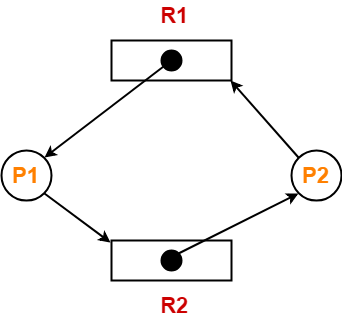Find if the system is in a deadlock state otherwise find a safe sequence.

## Solution-

### Method-01:

• The given resource allocation graph is single instance with a cycle contained in it.
• Thus, the system is definitely in a deadlock state.

### Method-02:

Using the given resource allocation graph, we have-

 Allocation Need R1 R2 R1 R2 Process P1 1 0 0 1 Process P2 0 1 1 0

Available = [ R1 R2 ] = [ 0 0 ]

Now,

• There are no instances available currently and both the processes require a resource to execute.
• Therefore, none of the process can be executed and both keeps waiting infinitely.
• Thus, the system is in a deadlock state.

## Problem-02:

Consider the resource allocation graph in the figure-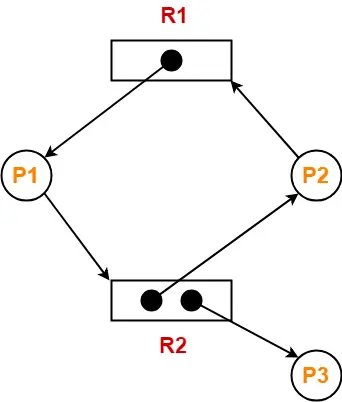Find if the system is in a deadlock state otherwise find a safe sequence.

## Solution-

• The given resource allocation graph is multi instance with a cycle contained in it.
• So, the system may or may not be in a deadlock state.

Using the given resource allocation graph, we have-

 Allocation Need R1 R2 R1 R2 Process P1 1 0 0 1 Process P2 0 1 1 0 Process P3 0 1 0 0

Available = [ R1 R2 ] = [ 0 0 ]

### Step-01:

• Since process P3 does not need any resource, so it executes.
• After execution, process P3 release its resources.

Then,

Available

= [ 0 0 ] + [ 0 1 ]

= [ 0 1 ]

### Step-02:

• With the instances available currently, only the requirement of the process P1 can be satisfied.
• So, process P1 is allocated the requested resources.
• It completes its execution and then free up the instances of resources held by it.

Then-

Available

= [ 0 1 ] + [ 1 0 ]

= [ 1 1 ]

### Step-03:

• With the instances available currently, the requirement of the process P2 can be satisfied.
• So, process P2 is allocated the requested resources.
• It completes its execution and then free up the instances of resources held by it.

Then-

Available

= [ 1 1 ] + [ 0 1 ]

= [ 1 2 ]

Thus,

• There exists a safe sequence P3, P1, P2 in which all the processes can be executed.
• So, the system is in a safe state.

## Problem-03:

Consider the resource allocation graph in the figure-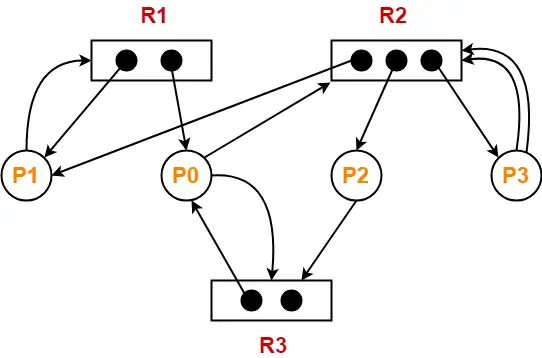Find if the system is in a deadlock state otherwise find a safe sequence.

## Solution-

• The given resource allocation graph is multi instance with a cycle contained in it.
• So, the system may or may not be in a deadlock state.

Using the given resource allocation graph, we have-

 Allocation Need R1 R2 R3 R1 R2 R3 Process P0 1 0 1 0 1 1 Process P1 1 1 0 1 0 0 Process P2 0 1 0 0 0 1 Process P3 0 1 0 0 2 0

Available = [ R1 R2 R3 ] = [ 0 0 1 ]

### Step-01:

• With the instances available currently, only the requirement of the process P2 can be satisfied.
• So, process P2 is allocated the requested resources.
• It completes its execution and then free up the instances of resources held by it.

Then-

Available

= [ 0 0 1 ] + [ 0 1 0 ]

= [ 0 1 1 ]

### Step-02:

• With the instances available currently, only the requirement of the process P0 can be satisfied.
• So, process P0 is allocated the requested resources.
• It completes its execution and then free up the instances of resources held by it.

Then-

Available

= [ 0 1 1 ] + [ 1 0 1 ]

= [ 1 1 2 ]

### Step-03:

• With the instances available currently, only the requirement of the process P1 can be satisfied.
• So, process P1 is allocated the requested resources.
• It completes its execution and then free up the instances of resources held by it.

Then-

Available

= [ 1 1 2 ] + [ 1 1 0 ]

= [ 2 2 2 ]

### Step-04:

• With the instances available currently, the requirement of the process P3 can be satisfied.
• So, process P3 is allocated the requested resources.
• It completes its execution and then free up the instances of resources held by it.

Then-

Available

= [ 2 2 2 ] + [ 0 1 0 ]

= [ 2 3 2 ]

Thus,

• There exists a safe sequence P2, P0, P1, P3 in which all the processes can be executed.
• So, the system is in a safe state.

To watch video solutions and practice other problems,

Watch this Video Lecture

Next Article- Contiguous Memory Allocation

Get more notes and other study material of Operating System.

Watch video lectures by visiting our YouTube channel LearnVidFun.

## Resource Allocation Graph-

 Resource Allocation Graph (RAG) is a graph that represents the state of a system pictorially.

It gives complete information about the state of a system such as-

• How many processes exist in the system?
• How many instances of each resource type exist?
• How many instances of each resource type are allocated?
• How many instances of each resource type are still available?
• How many instances of each resource type are held by each process?
• How many instances of each resource type does each process need for execution?

## Components Of RAG-

There are two major components of a Resource Allocation Graph-

1. Vertices
2. Edges

## Vertices-

There are following types of vertices in a Resource Allocation Graph-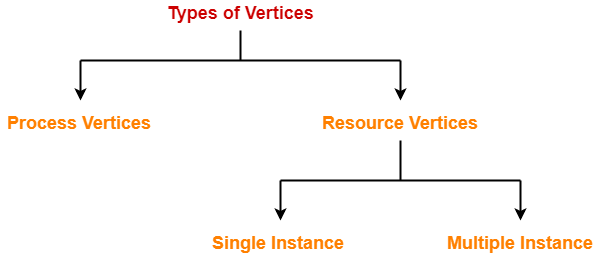1. Process Vertices
2. Resource Vertices

## Process Vertices-

• Process vertices represent the processes.
• They are drawn as a circle by mentioning the name of process inside the circle.

## Resource Vertices-

• Resource vertices represent the resources.
• Depending on the number of instances that exists in the system, resource vertices may be single instance or multiple instance.
• They are drawn as a rectangle by mentioning the dots inside the rectangle.
• The number of dots inside the rectangle indicates the number of instances of that resource existing in the system.

## Edges-

There are two types of edges in a Resource Allocation Graph-1. Assign Edges
2. Request Edges

## Assign Edges-

• Assign edges represent the assignment of resources to the processes.
• They are drawn as an arrow where the head of the arrow points to the process and tail of the process points to the instance of the resource.

## Request Edges-

• Request edges represent the waiting state of processes for the resources.
• They are drawn as an arrow where the head of the arrow points to the instance of the resource and tail of the process points to the process.
• If a process requires ‘n’ instances of a resource type, then ‘n’ assign edges will be drawn.

## Example Of RAG-

The following diagram represents a Resource Allocation Graph-It gives the following information-

• There exist three processes in the system namely P1, P2 and P3.
• There exist two resources in the system namely R1 and R2.
• There exists a single instance of resource R1 and two instances of resource R2.
• Process P1 holds one instance of resource R1 and is waiting for an instance of resource R2.
• Process P2 holds one instance of resource R2 and is waiting for an instance of resource R1.
• Process P3 holds one instance of resource R2 and is not waiting for anything.

To gain better understanding about Resource Allocation Graph,

Watch this Video Lecture

Next Article- Practice Problems On Resource Allocation Graph

Get more notes and other study material of Operating System.

Watch video lectures by visiting our YouTube channel LearnVidFun.

Before you go through this article, make sure that you have gone through the previous article on Deadlock in OS.

We have discussed-

• In a deadlock state, the execution of multiple processes is blocked.
• There are 4 necessary conditions for the occurrence of deadlock.

## Important Concept-

Consider there are n processes in the system P1, P2, P3, …… , Pn where-

• Process P1 requires x1 units of resource R
• Process P2 requires x2 units of resource R
• Process P3 requires x3 units of resource R and so on.

In worst case,

 The number of units that each process holds = One less than its maximum demand

So,

• Process P1 holds x1 – 1 units of resource R
• Process P2 holds x2 – 1 units of resource R
• Process P3 holds x3 – 1 units of resource R and so on.

Now,

• Had there been one more unit of resource R in the system, system could be ensured deadlock free.
• This is because that unit would be allocated to one of the processes and it would get execute and then release its units.

From here, we have-

### Maximum Number Of Units That Ensures Deadlock-

Maximum number of units of resource R that ensures deadlock

= (x1-1) + (x2-1) + (x3-1) + …. + (xn-1)

= ( x1 + x2 + x3 + …. + xn ) – n

= ∑xi – n

= Sum of max needs of all n processes – n

### Minimum Number Of Units That Ensures No Deadlock-

Minimum number of units of resource R that ensures no deadlock

= One more than maximum number of units of resource R that ensures deadlock

= (∑xi – n) + 1

## Problem-01:

A system is having 3 user processes each requiring 2 units of resource R. The minimum number of units of R such that no deadlock will occur-

1. 3
2. 5
3. 4
4. 6

## Solution-

In worst case,

The number of units that each process holds = One less than its maximum demand

So,

• Process P1 holds 1 unit of resource R
• Process P2 holds 1 unit of resource R
• Process P3 holds 1 unit of resource R

Thus,

• Maximum number of units of resource R that ensures deadlock = 1 + 1 + 1 = 3
• Minimum number of units of resource R that ensures no deadlock = 3 + 1 = 4

## Problem-02:

A system is having 10 user processes each requiring 3 units of resource R. The minimum number of units of R such that no deadlock will occur _____?

## Solution-

In worst case,

The number of units that each process holds = One less than its maximum demand

So,

• Process P1 holds 2 units of resource R
• Process P2 holds 2 units of resource R
• Process P3 holds 2 units of resource R and so on.
• Process P10 holds 2 units of resource R

Thus,

• Maximum number of units of resource R that ensures deadlock = 10 x 2 = 20
• Minimum number of units of resource R that ensures no deadlock = 20 + 1 = 21

## Problem-03:

A system is having 3 user processes P1, P2 and P3 where P1 requires 2 units of resource R, P2 requires 3 units of resource R, P3 requires 4 units of resource R. The minimum number of units of R that ensures no deadlock is _____?

## Solution-

In worst case,

The number of units that each process holds = One less than its maximum demand

So,

• Process P1 holds 1 unit of resource R
• Process P2 holds 2 units of resource R
• Process P3 holds 3 units of resource R

Thus,

• Maximum number of units of resource R that ensures deadlock = 1 + 2 + 3 = 6
• Minimum number of units of resource R that ensures no deadlock = 6 + 1 = 7

## Problem-04:

A system is having 3 user processes P1, P2 and P3 where P1 requires 21 units of resource R, P2 requires 31 units of resource R, P3 requires 41 units of resource R. The minimum number of units of R that ensures no deadlock is _____?

## Solution-

In worst case,

The number of units that each process holds = One less than its maximum demand

So,

• Process P1 holds 20 units of resource R
• Process P2 holds 30 units of resource R
• Process P3 holds 40 units of resource R

Thus,

• Maximum number of units of resource R that ensures deadlock = 20 + 30 + 40 = 90
• Minimum number of units of resource R that ensures no deadlock = 90 + 1 = 91

## Problem-05:

If there are 6 units of resource R in the system and each process in the system requires 2 units of resource R, then how many processes can be present at maximum so that no deadlock will occur?

## Solution-

In worst case,

The number of units that each process holds = One less than its maximum demand

So,

• Process P1 holds 1 unit of resource R
• Process P2 holds 1 unit of resource R
• Process P3 holds 1 unit of resource R
• Process P4 holds 1 unit of resource R
• Process P5 holds 1 unit of resource R
• Process P6 holds 1 unit of resource R

Thus,

• Minimum number of processes that ensures deadlock = 6
• Maximum number of processes that ensures no deadlock = 6 – 1 = 5

## Problem-06:

If there are 6 units of resource R in the system and each process in the system requires 3 units of resource R, then how many processes can be present at maximum so that no deadlock will occur?

## Solution-

In worst case,

The number of units that each process holds = One less than its maximum demand

So,

• Process P1 holds 2 units of resource R
• Process P2 holds 2 units of resource R
• Process P3 holds 2 units of resource R

Thus,

• Minimum number of processes that ensures deadlock = 3
• Maximum number of processes that ensures no deadlock = 3 – 1 = 2

## Problem-07:

If there are 100 units of resource R in the system and each process in the system requires 2 units of resource R, then how many processes can be present at maximum so that no deadlock will occur?

## Solution-

In worst case,

The number of units that each process holds = One less than its maximum demand

So,

• Process P1 holds 1 unit of resource R
• Process P2 holds 1 unit of resource R
• Process P3 holds 1 unit of resource R and so on.
• Process P100 holds 1 unit of resource R

Thus,

• Minimum number of processes that ensures deadlock = 100
• Maximum number of processes that ensures no deadlock = 100 – 1 = 99

## Problem-08:

If there are 100 units of resource R in the system and each process in the system requires 4 units of resource R, then how many processes can be present at maximum so that no deadlock will occur?

## Solution-

In worst case,

The number of units that each process holds = One less than its maximum demand

So,

• Process P1 holds 3 units of resource R
• Process P2 holds 3 units of resource R
• Process P3 holds 3 units of resource R and so on.
• Process P33 holds 3 units of resource R
• Process P34 holds 1 unit of resource R

Thus,

• Minimum number of processes that ensures deadlock = 34
• Maximum number of processes that ensures no deadlock = 34 – 1 = 33

## Problem-09:

If there are 100 units of resource R in the system and each process in the system requires 5 units of resource R, then how many processes can be present at maximum so that no deadlock will occur?

## Solution-

In worst case,

The number of units that each process holds = One less than its maximum demand

So,

• Process P1 holds 4 units of resource R
• Process P2 holds 4 units of resource R
• Process P3 holds 4 units of resource R and so on.
• Process P25 holds 4 units of resource R

Thus,

• Minimum number of processes that ensures deadlock = 25
• Maximum number of processes that ensures no deadlock = 25 – 1 = 24

## Problem-10:

A computer system has 6 tape drives with n processes competing for them. Each process needs 3 tape drives. The maximum value of n for which the system is guaranteed to be deadlock free-

1. 2
2. 3
3. 4
4. 1

## Solution-

In worst case,

The number of tape drives that each process holds = One less than its maximum demand

So,

• Process P1 holds 2 tape drives
• Process P2 holds 2 tape drives
• Process P3 holds 2 tape drives

Thus,

• Minimum number of processes that ensures deadlock = 3
• Maximum number of processes that ensures no deadlock = 3 – 1 = 2

## Problem-11:

Consider a system having m resources of the same type. These resources are shared by 3 processes A, B and C which have peak demands of 3, 4 and 6 respectively. For what value of m, deadlock will not occur?

1. 7
2. 9
3. 10
4. 13

## Solution-

In worst case,

The number of units that each process holds = One less than its maximum demand

So,

• Process A holds 2 units of resource R
• Process B holds 3 units of resource R
• Process C holds 5 units of resource R

Thus,

• Maximum number of units of resource R that ensures deadlock = 2 + 3 + 5 = 10
• Minimum number of units of resource R that ensures no deadlock = 10 + 1 = 11

So, any number of units greater than 11 will ensure no deadlock.

Thus, Option (D) is correct.

## Problem-12:

Consider a system having m resources of the same type being shared by n processes. Resources can be requested and released by processes only one at a time. The system is deadlock free if and only if-

1. The sum of all max needs is < m+n
2. The sum of all max needs is > m+n
3. Both of above
4. None of these

## Solution-

We have derived above-

Maximum number of units of resource R that ensures deadlock = (∑xi – n)

Number of units of resource R must be > (∑xi – n)

i.e. m > (∑xi – n)

or ∑xi < m + n

Thus, Correct Option is (A).

## Problem-13:

Consider the following snapshot of a system running n processes. Process i is holding xi instances of a resource R for 1<=i<=n. Currently, all instances of R are occupied. Further, for all i, process i has placed a request for an additional yi instances while holding the xi instances it already has. There are exactly two processes p and q such that yp = yq = 0. Which of the following can serve as a necessary condition to guarantee that the system is not approaching a deadlock?

1. min(xp, xq) < maxk≠p,qyk
2. xp + xq >= mink≠p,qyk
3. min(xp, xq) < 1
4. min(xp, xq) >1

## Solution-

According to question, we have-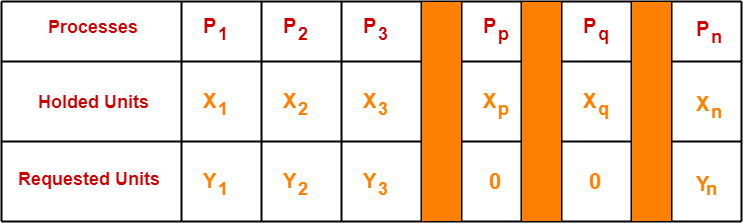• Clearly, processes Pp and Pq do not require any additional resource.
• So they continue their execution.
• After getting executed completely, they release the units allocated to them.
• Thus, the total units that get free up = xp + xq

Now,

• To ensure that other processes are executed without any deadlock, the total amount of units freely available currently ( xp + xq ) must be able to meet the requirements of some other process.
• If available ( xp + xq ) units could not meet the requirement of any other process, then certainly there would be deadlock.

Thus, for no deadlock, the necessary condition is-

xp + xq >= min yk where k ≠ p, q

Thus, Correct Option is (B).

### NOTE-

• It is very important to note that the above condition is just a necessary condition and not at all a sufficient condition to avoid the deadlock.
• The above condition just ensures that the system is able to proceed from the current state.
• It does not guarantee that there won’t be a deadlock before all the other processes are finished.
• The sufficient condition to avoid the deadlock would be either xp + xq >= ∑ yi or xp + xq >= max yk where k ≠ p, q.

To watch video solutions and practice other problems,

Watch this Video Lecture

Next Article- Banker’s Algorithm

Get more notes and other study material of Operating System.

Watch video lectures by visiting our YouTube channel LearnVidFun.

Before you go through this article, make sure that you have gone through the previous article on Deadlock in OS.

The various strategies for handling deadlock are-• This strategy involves designing a system that violates one of the four necessary conditions required for the occurrence of deadlock.
• This ensures that the system remains free from the deadlock.

The various conditions of deadlock occurrence may be violated as-

## 1. Mutual Exclusion-

• To violate this condition, all the system resources must be such that they can be used in a shareable mode.
• In a system, there are always some resources which are mutually exclusive by nature.
• So, this condition can not be violated.

## 2. Hold and Wait-

This condition can be violated in the following ways-

### Approach-01:

In this approach,

• A process has to first request for all the resources it requires for execution.
• Once it has acquired all the resources, only then it can start its execution.
• This approach ensures that the process does not hold some resources and wait for other resources.

### Drawbacks-

The drawbacks of this approach are-

• It is less efficient.
• It is not implementable since it is not possible to predict in advance which resources will be required during execution.

### Approach-02:

In this approach,

• A process is allowed to acquire the resources it desires at the current moment.
• After acquiring the resources, it start its execution.
• Now before making any new request, it has to compulsorily release all the resources that it holds currently.
• This approach is efficient and implementable.

### Approach-03:

In this approach,

• A timer is set after the process acquires any resource.
• After the timer expires, a process has to compulsorily release the resource.

## 3. No Preemption-

• This condition can by violated by forceful preemption.
• Consider a process is holding some resources and request other resources that can not be immediately allocated to it.
• Then, by forcefully preempting the currently held resources, the condition can be violated.

 A process is allowed to forcefully preempt the resources possessed by some other process only if- It is a high priority process or a system process. The victim process is in the waiting state.

## 4. Circular Wait-

• This condition can be violated by not allowing the processes to wait for resources in a cyclic manner.
• To violate this condition, the following approach is followed-

### Approach-

• A natural number is assigned to every resource.
• Each process is allowed to request for the resources either in only increasing or only decreasing order of the resource number.
• In case increasing order is followed, if a process requires a lesser number resource, then it must release all the resources having larger number and vice versa.
• This approach is the most practical approach and implementable.
• However, this approach may cause starvation but will never lead to deadlock.

• This strategy involves maintaining a set of data using which a decision is made whether to entertain the new request or not.
• If entertaining the new request causes the system to move in an unsafe state, then it is discarded.
• This strategy requires that every process declares its maximum requirement of each resource type in the beginning.
• The main challenge with this approach is predicting the requirement of the processes before execution.
• Banker’s Algorithm is an example of a deadlock avoidance strategy.

• This strategy involves waiting until a deadlock occurs.
• After deadlock occurs, the system state is recovered.
• The main challenge with this approach is detecting the deadlock.

• This strategy involves ignoring the concept of deadlock and assuming as if it does not exist.
• This strategy helps to avoid the extra overhead of handling deadlock.
• Windows and Linux use this strategy and it is the most widely used method.
• It is also called as Ostrich approach.

Watch this Video Lecture

Next Article- Practice Problems On Deadlock

Get more notes and other study material of Operating System.

Watch video lectures by visiting our YouTube channel LearnVidFun.

• The execution of two or more processes is blocked because each process holds some resource and waits for another resource held by some other process.

## Example-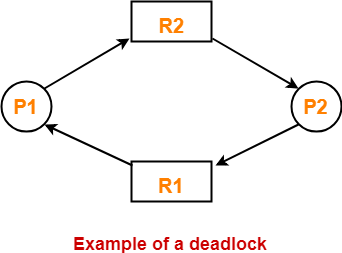Here

• Process P1 holds resource R1 and waits for resource R2 which is held by process P2.
• Process P2 holds resource R2 and waits for resource R1 which is held by process P1.
• None of the two processes can complete and release their resource.
• Thus, both the processes keep waiting infinitely.

There are following 4 necessary conditions for the occurrence of deadlock-

1. Mutual Exclusion
2. Hold and Wait
3. No preemption
4. Circular wait

## 1. Mutual Exclusion-

By this condition,

• There must exist at least one resource in the system which can be used by only one process at a time.
• If there exists no such resource, then deadlock will never occur.
• Printer is an example of a resource that can be used by only one process at a time.

## 2. Hold and Wait-

By this condition,

• There must exist a process which holds some resource and waits for another resource held by some other process.

## 3. No Preemption-

By this condition,

• Once the resource has been allocated to the process, it can not be preempted.
• It means resource can not be snatched forcefully from one process and given to the other process.
• The process must release the resource voluntarily by itself.

## 4. Circular Wait-

By this condition,

• All the processes must wait for the resource in a cyclic manner where the last process waits for the resource held by the first process.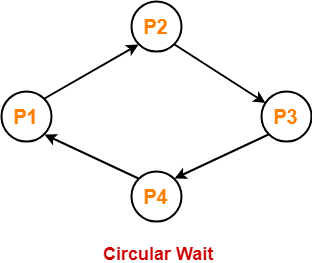Here,

• Process P1 waits for a resource held by process P2.
• Process P2 waits for a resource held by process P3.
• Process P3 waits for a resource held by process P4.
• Process P4 waits for a resource held by process P1.

## Important Note-

• All these 4 conditions must hold simultaneously for the occurrence of deadlock.
• If any of these conditions fail, then the system can be ensured deadlock free.